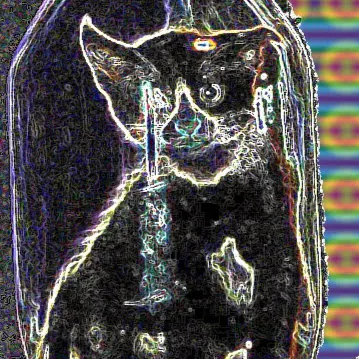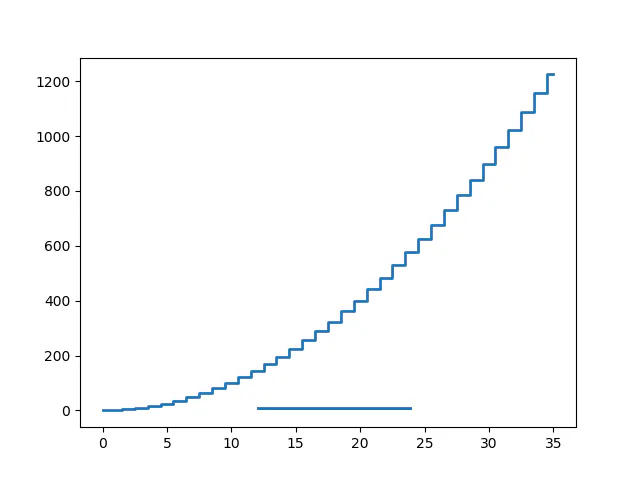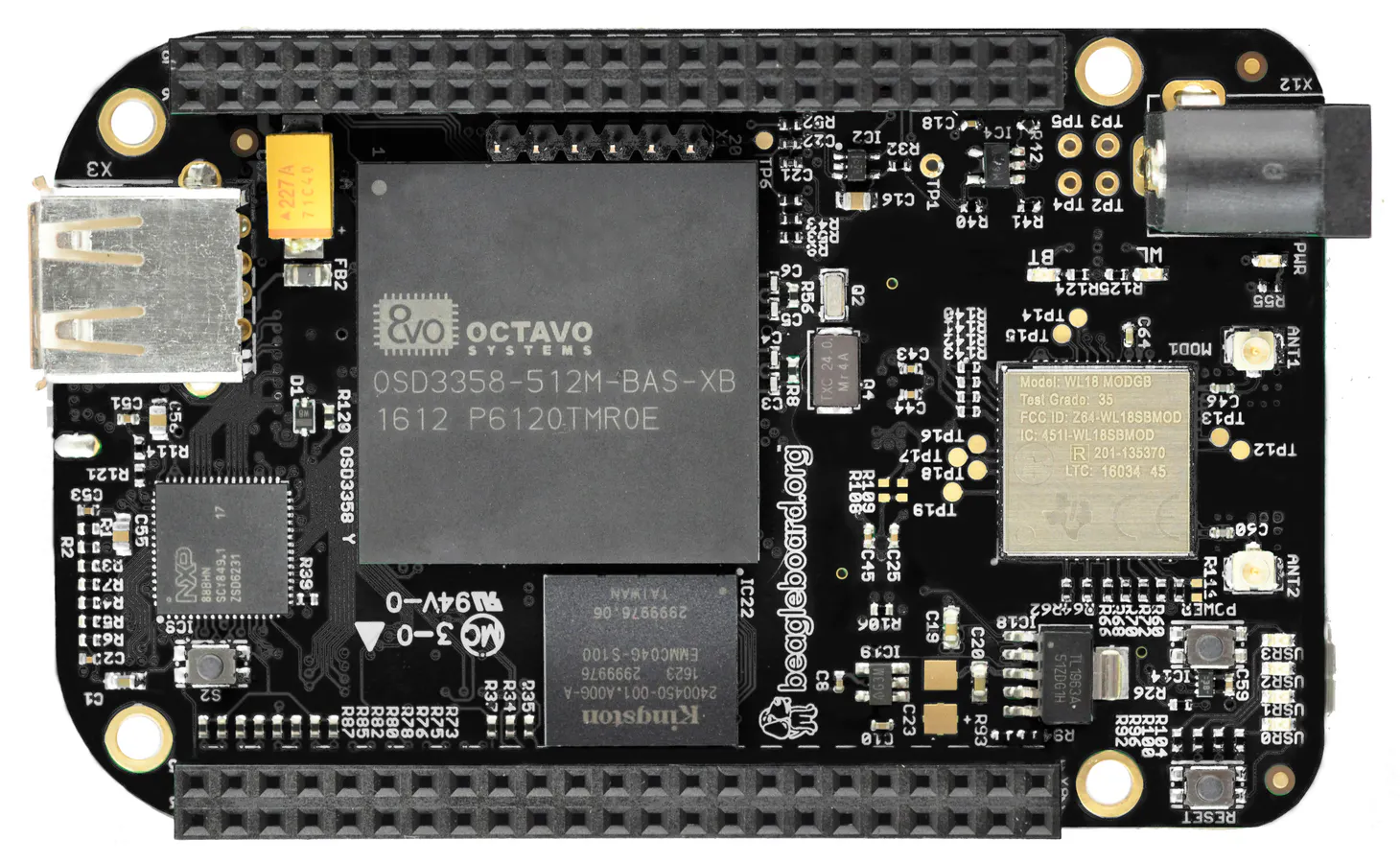Published

# Matplotlib on the BeagleBone Black Wireless

Are you interested in visual plotting for statistics? Matplotlib!

BeginnerProtip5 hours49## Things used in this project

### Hardware componentsBeagleBoard.org BeagleBone Black Wireless
×1

### Software apps and online services

 Matplotlib
 Python

Here it is!

## Code

### Source

Python
Enjoy!
```from mpl_toolkits import mplot3d
import numpy as np
from matplotlib import pyplot as plt, patches
import os

def main():
path = "molt"
_fig, ax = plt.subplots()
for i in range(16):
ax = plt.axes(projection="3d")
zline = np.linspace(0, 15, 1000)
xline = np.sin(zline)
yline = np.cos(zline)
ax.plot3D(xline, yline, zline, "red")
zdata = 15 * np.random.random(100)
xdata = np.sin(zdata) + 0.1 * np.random.randn(100)
ydata = np.cos(zdata) + 0.1 * np.random.randn(100)
ax.scatter3D(xdata, ydata, zdata, c=zdata, cmap="Greens")
figname = "fig_{}.png".format(i)
dest = os.path.join(path, figname)
plt.savefig(dest)
plt.cla()
print("Completed!")

main()
```

## Credits

### Mala Dies

11 projects • 1 follower
Stay there and someone will find you...
Thanks to Don Kirby.